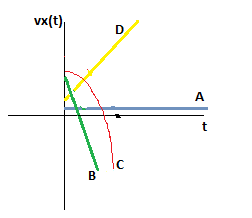# Which of the curves labeled A to D on the graph below could be a plot of v_x(t), the velocity of...

## Question:

Which of the curves labeled A to D on the graph below could be a plot of {eq}v_x(t) {/eq}, the velocity of the object in the x direction as a function of time?## Velocity time graph:

Velocity-time graphs are used to study about the acceleration with the help of slope, as value of slope in v-t graph is numerically equal to the acceleration.

## Answer and Explanation:

{eq}\text{Let initially, the object is moving with some velocity $v_o$}\\ \text{the velocity of the object gradually decreases due to negative...

See full answer below.

Become a Study.com member to unlock this answer! Create your account

#### Learn more about this topic:Using Velocity vs. Time Graphs to Describe Motion

from AP Physics 1: Exam Prep

Chapter 3 / Lesson 14
115K